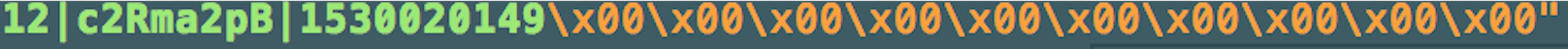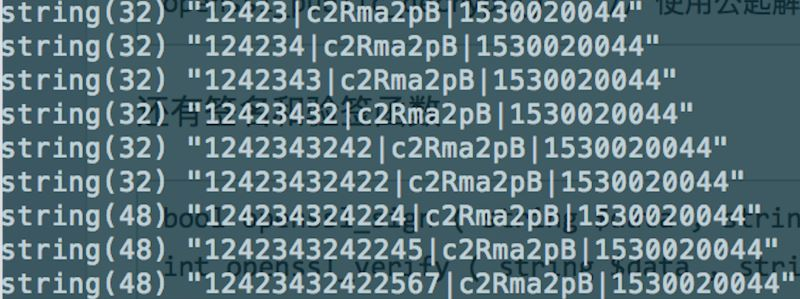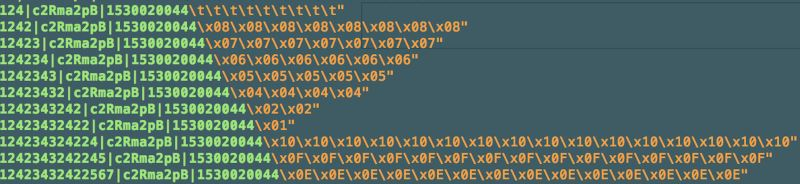# PHP7.1中使用openssl替换mcrypt的实例详解

mcrypt未使用填充

mcrypt加密:

``` \$key = 'aaaaaaaaaaaaaaaaaaaaaaaaaaaaaa';
\$iv = 'aaaaaaaaaaaaaaaa';
\$data = 'dataString';
\$cipher = mcrypt_module_open(MCRYPT_RIJNDAEL_128, '', MCRYPT_MODE_CBC, '');
mcrypt_generic_init(\$cipher, \$key, \$iv);
\$cipherText256 = mcrypt_generic(\$cipher, \$data);
mcrypt_generic_deinit(\$cipher);
return bin2hex(\$cipherText256);```

``` \$key = 'aaaaaaaaaaaaaaaaaaaaaaaaaaaaaa';
\$iv = 'aaaaaaaaaaaaaaaa';
\$data = 'dataString';```
``` \$data = \$data . str_repeat("\x00", 16 - (strlen(\$data) % 16)); // 双引号可以解析asc-ii码\x00
return bin2hex(openssl_encrypt(\$data, "AES-256-CBC", \$key, OPENSSL_RAW_DATA | OPENSSL_ZERO_PADDING, \$iv));```

mcrypt使用填充

mcrypt加密:

```\$key = 'aaaaaaaaaaaaaaaaaaaaaaaaaaaaaa';
\$iv = 'aaaaaaaaaaaaaaaa';
\$data = 'dataString';
// 填充（移除填充反着移除即可）
\$block = mcrypt_get_block_size(MCRYPT_RIJNDAEL_128, MCRYPT_MODE_CBC);
\$pad = \$block - (strlen(\$data) % \$block);
if (\$pad <= \$block) {
\$char = chr(\$pad);
\$data .= str_repeat(\$char, \$pad);
}
\$cipher = mcrypt_module_open(MCRYPT_RIJNDAEL_128, '', MCRYPT_MODE_CBC, '');
mcrypt_generic_init(\$cipher, \$key, \$iv);
\$cipherText256 = mcrypt_generic(\$cipher, \$data);
mcrypt_generic_deinit(\$cipher);
return bin2hex(\$cipherText256);```

``` \$key = 'aaaaaaaaaaaaaaaaaaaaaaaaaaaaaa';
\$iv = 'aaaaaaaaaaaaaaaa';
\$data = 'dataString';
return bin2hex(openssl_encrypt(\$data, 'AES-256-CBC', \$key, OPENSSL_RAW_DATA | OPENSSL_ZERO_PADDING, \$iv));```

mcrypt默认填充mcrypt默认填充

``` // 尾部包含若干个`\x00` 均可功输出true
if ("string\x00" == "string") { // 用双引号可解析\x00
echo true;
}```

\x00填充后的示例：(请注意字符串的长度，由此可见用\x00填充会影响长度)mcrypt自主填充

```/**
* 填充算法
* @param string \$source
* @return string
*/
function addPKCS7Padding(\$source)
{
\$source = trim(\$source);
\$block = mcrypt_get_block_size(MCRYPT_RIJNDAEL_128, MCRYPT_MODE_CBC);

\$pad = \$block - (strlen(\$source) % \$block);
if (\$pad <= \$block) {
\$char = chr(\$pad);
\$source .= str_repeat(\$char, \$pad);
}
return \$source;
}
`````` /**
* 移去填充算法
* @param string \$source
* @return string
*/
function stripPKSC7Padding(\$source)
{
\$source = trim(\$source);
\$char = substr(\$source, -1);
\$num = ord(\$char);
if (\$num == 62) return \$source;
\$source = substr(\$source, 0, -\$num);
return \$source;
}```

openssl默认填充openssl自主填充

openssl_encrypt提供了option参数以支持自主填充，但在查阅php源码中openssl的测试用例代码才找到正确用法:

``` // if we want to manage our own padding
\$padded_data = \$data . str_repeat(' ', 16 - (strlen(\$data) % 16));
\$encrypted = openssl_encrypt(\$padded_data, \$method, \$password, OPENSSL_RAW_DATA|OPENSSL_ZERO_PADDING, \$iv);
\$output = openssl_decrypt(\$encrypted, \$method, \$password, OPENSSL_RAW_DATA|OPENSSL_ZERO_PADDING, \$iv);
var_dump(rtrim(\$output));
（备注：如上，OPENSSL_ZERO_PADDING 并非是为0填充的意思）```

mcrypt加密时未使用填充，故以\x00进行了填充，所以在替换成openssl，需要自主实现\x00填充。

mcrypt加密时使用了标准的填充，同时openssl的填充方式也为标准填充，故直接使用即可。